# Thoughts on Bubble Plots...

July 19, 2018

I have had a lot of fun making cool plots and designing their geometry from scratch because it offers a lot of interesting ideas both to understand OOP and geometry/math.

In this blog, I would share you the whole thought process so that you too can make plots like that.

Let’s start with the textbook definition of bubble plot A bubble chart is a type of chart that displays three dimensions of data. Each entity with its triplet (v1, v2, v3) of associated data is plotted as a disk that expresses two of the vi values through the disk’s xy location and the third through its size.

Practically, we can think of Bubble plot as a visualization that helps compare the intensity of data-points in a 2d space. For example let’s look at a very classic bubble plot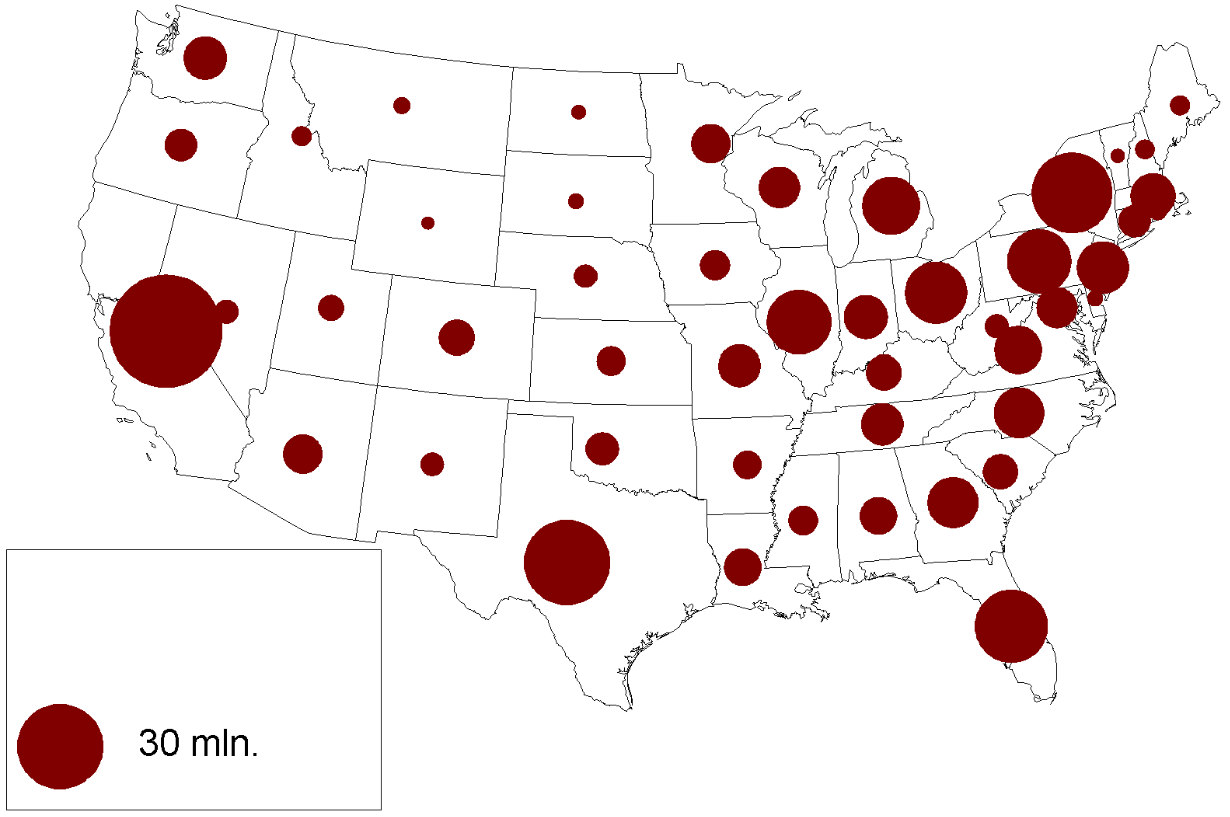Here we have a 2-d space that shows a map of the united states and the diameter of different circles helps us compare the population in different states. This clearly establishes the relevance of bubble plot as a plotting mechanism that helps compare values in a 2-d space. This idea can also be mapped to many other plotting tasks

Now that we know why of making bubble plots lets move to the how by looking at Rubyplot codebase.

The whole bubble class code base is divided into 3 files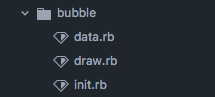init.rb -> Initialization the bubble class
draw.rb -> Compilation of drawing functions
data.rb -> Operations to get geometry information from the user data

Overall, I have made a simple bubble class that inherits from scatter plot because scatter plot and bubble plot are very similar at their core ideas. To get into the inner runnings lets run some code and explore how it works.

``````plot = Rubyplot::Bubble.new
plot.data(:apples, [-1, 19, -4, -23], [-35, 21, 23, -4], [45, 10, 21, 9])
plot.data(:peaches, [20, 30, -6, -3], [-1, 5, -27, -3], [13, 10, 20, 10])
plot.set_colors_array(%w[white yellow])
plot.write('spec/reference_images/bubble.png')
``````

This code is implemented internally in the library as ->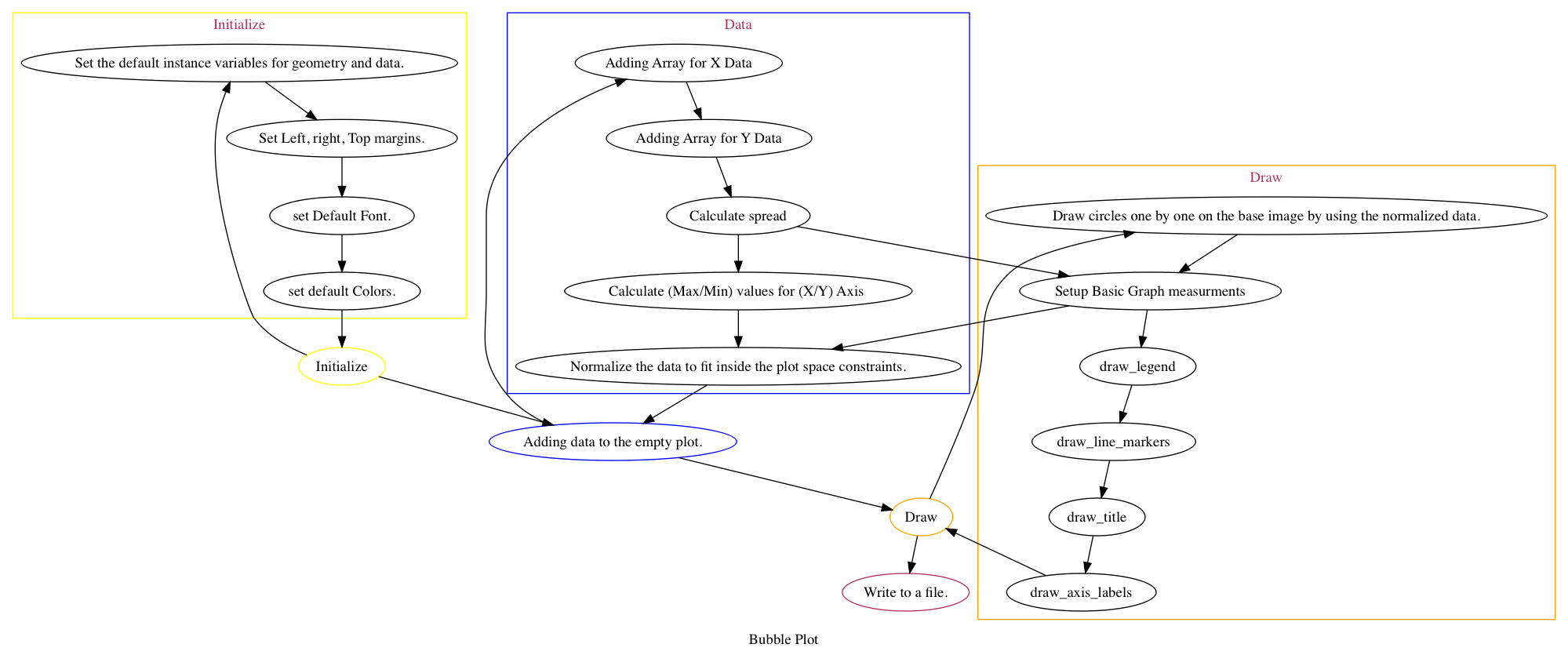Now let’s walk through the codebase

1. Initialization -> we start off initially with the setting up of a few default background colors, fonts etc can be set at the start.
``````plot = Rubyplot::Bubble.new
``````
2. Data Addition -> Data is added in set of three arrays
``````plot.data(:apples, [-1, 19, -4, -23], [-35, 21, 23, -4], [45, 10, 21, 9])
plot.data(:peaches, [20, 30, -6, -3], [-1, 5, -27, -3], [13, 10, 20, 10])
``````

These arrays represent the X, Y co-ordinate and the proportional circle radius in the third array. At first there is the spread calculation along all the arrays to get the maximum/minimum values for X/Y Axis and this helps to figure out how to best give enough space to the bubbles made in the plot. Notice that the spread calculation involves taking the maximums/minimum values of the the arrays after doing addition/subtraction between X/Y and the Z axis. This is because the spread the depends not only on the co-ordinates of an individual bubble but also on the size of the bubble itself. Taking this into account solves the problem.

3. `plot.set_colors_array(%w[white yellow])`
This function just sets the colors for every single label of the graph. It’s pretty cool but it’s not absolutely necessary to use this function. When the user doesn’t set this function the colors are automatically selected randomly from the list of colors supported by RMAgick.

4. `plot.write('spec/reference_images/bubble.png')` Finally this function call does the whole sorcery of RubyPlot.

A. At first it sets up the drawing by first calculating the data spread to normalize the data and then setting up the all the basic geometry measurements of the graph.

B. Then it sets up the graph pixel boundaries from all the sides like right, top, left, bottom and it also sets the label heights boundaries and space offsets. So until this part it makes the calculations that will eventually make the graph.

C. The actual drawing process starts where it writes the legends, title and the axis labels.
At this point our graph is basically empty and looks like this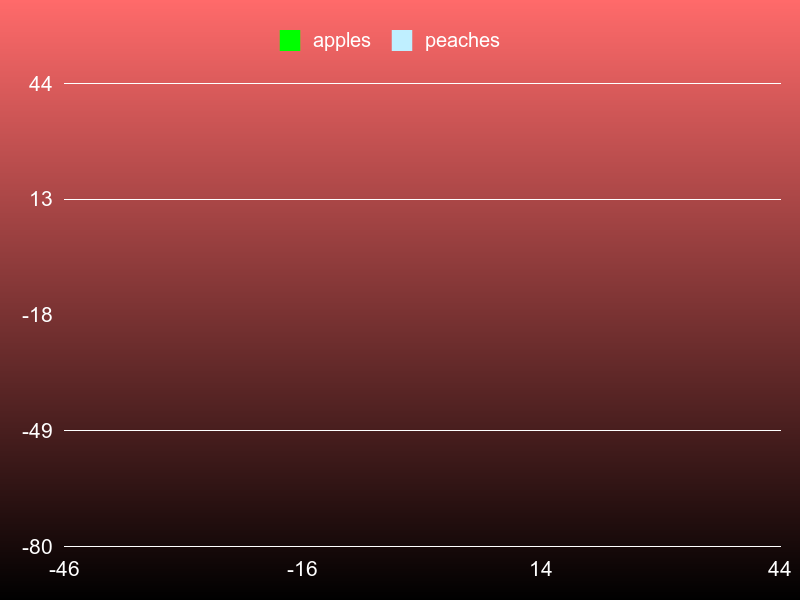D. It is then that the normalized data is finally plotted one by one in the form of circles on the plot the code is simple and easy to understand.

You can watch the evolution of a plot with it’s data in these sequences of images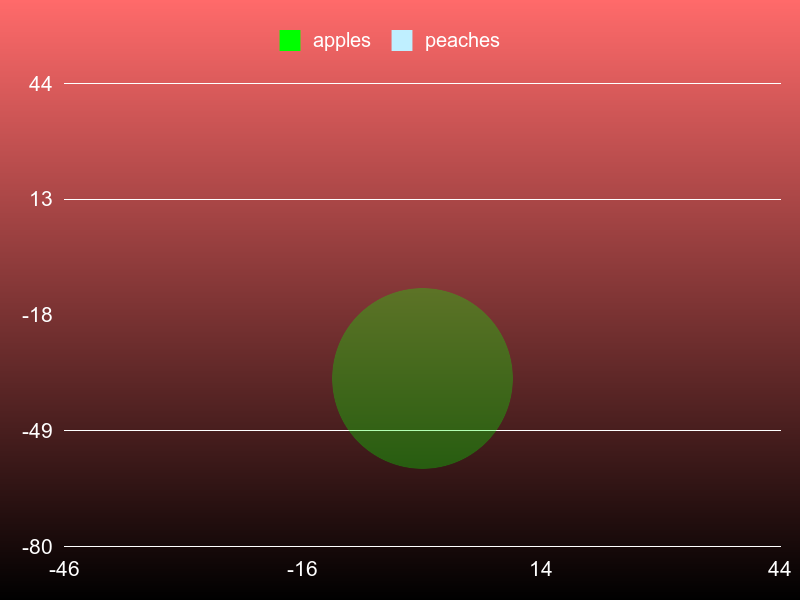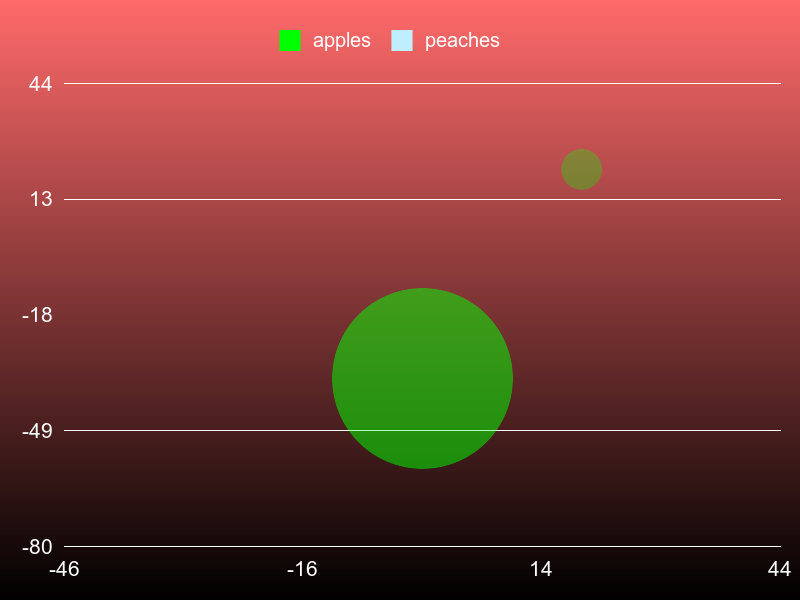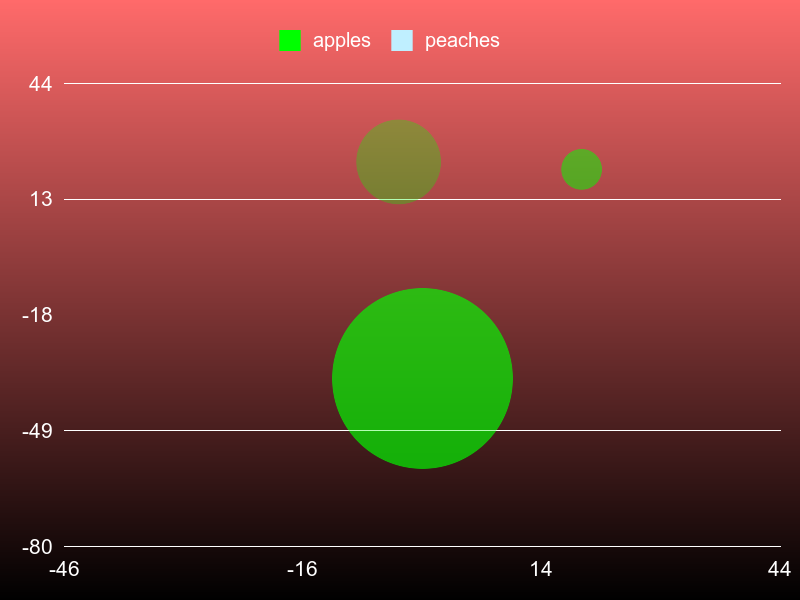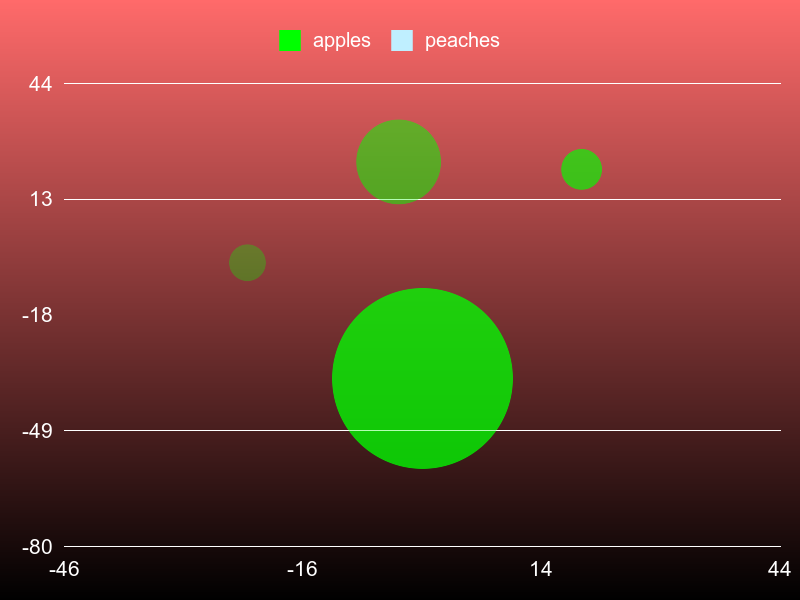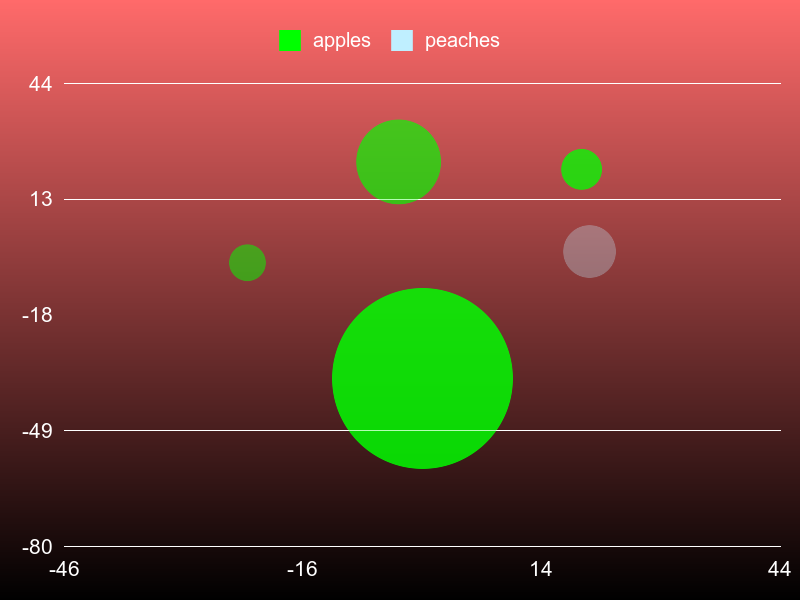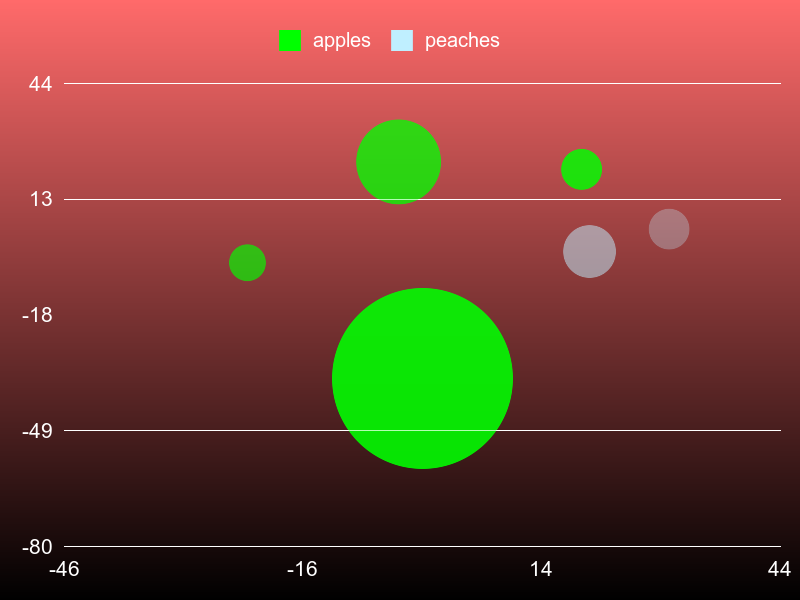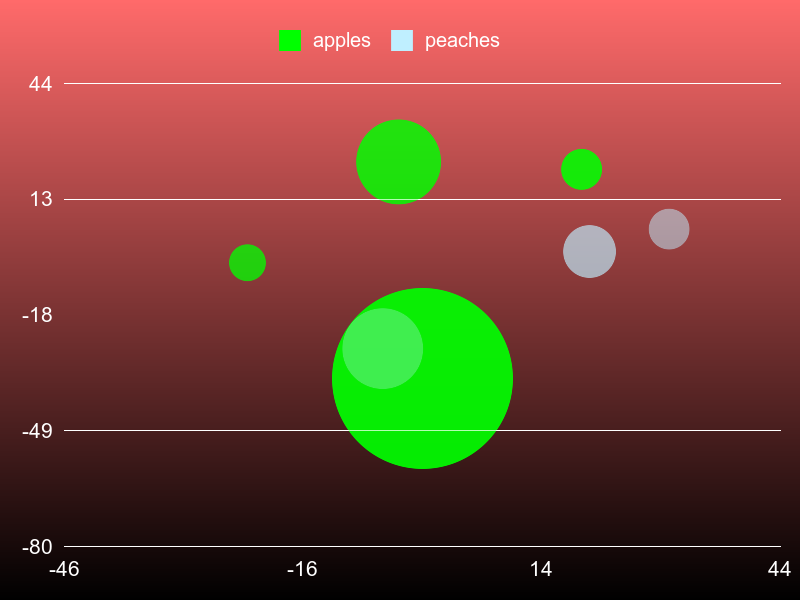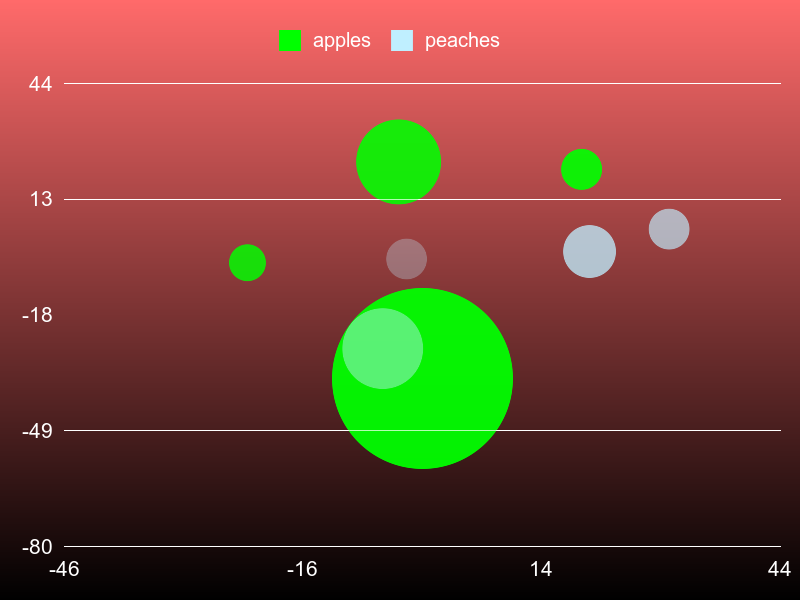It shows how the plot is “filled up” as the loops proceeds. On the completion of the process we have a clean and beautiful bubble plot for our data.

Thanks a lot for reading this story… I hope you learn something from this and make something cool of your own.